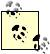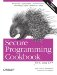# Using Hashed Containers

Problem

You are storing keys and values , you need constant-time access to elements, and you don't need the elements to be stored in sorted order.

Solution

Use one of the hashed associated containers, hash_map or hash_set. Be aware, however, that these are not standard containers specified by the C++ Standard, rather they are extensions that most standard library implementations include. Example 6-8 shows how to use a hash_set.

Example 6-8. Storing strings in a hash_set

```#include
#include
#include

int main( ) {

hash_set hsString;
string s = "bravo";

hsString.insert(s);
s = "alpha";
hsString.insert(s);
s = "charlie";
hsString.insert(s);

for (hash_set::const_iterator p = hsString.begin( );
p != hsString.end( ); ++p)
cout << *p << endl; // Note that these aren't guaranteed
// to be in sorted order
}```

Discussion

Hashed containers are popular data structures in any language, and it is unfortunate that C++ Standard does not require an implementation to supply them. All is not lost, however, if you want to use a hashed container: chances are that the standard library implementation you are using includes hash_map and hash_set, but the fact that they are not standardized means their interfaces may differ from one standard library implementation to the next. I will describe the hashed containers that are provided in the STLPort standard library implementation.STLPort is a free, portable standard library implementation that has been around for a long time and provides hashed containers. If you are using a different library, the interface may be different, but the general idea is the same.

The main characteristics of hashed containers (called hashed associative containers by much of the C++ literature) are that they provide, in the average case, constant-time location, insertion, and deletion of elements; in the worst case, operations require linear complexity. The trade-off for all of these constant-time operations is that the elements in a hashed container are not stored in order, as they are in a map.

Look at Example 6-8. Using a hashed container (in this case, a hash_set) is simple enoughdeclare it like most other containers and start inserting things into it:

```hash_set hsString; // A hash_set of strings
string s = "bravo";
hsString.insert(s); // Insert a copy of s```

Using a hash_map is similar, except that (minimally) you have to specify both the key and the data types that will be used. This is identical to a map:

```hash_map
hmStrings; // Map strings to strings
string key = "key";
string val = "val";
hmStrings[key] = val;```

These are just the basics of using hashed containers; there are a handful of additional template parameters that let you specify the hash function to use, the function to use to test for key equivalency, and an object to use for memory allocation. I discuss these a little later.

There are four hashed containers in most libraries, and they resemble the other associative containers in the standard library (i.e., map and set), they are hash_map, hash_multimap, hash_set, and hash_multiset. Hashed containers are all implemented using a hash table. A hash table is a data structure that allows constant-time access to elements by, basically, using a hash function to "jump" to a location close to where the desired object is stored instead of traversing through a tree-like structure. The difference between hash_map and hash_set is how the data are stored in the hash table.

The declarations for the hash table-based containers in STLPort are as follows:

```hash_map, // The hash function to use
EqualKey = equal_to, // Function to use for key
// equivalence test
Alloc = alloc> // The allocator to use

hash_set, // The hash function to use
EqualKey = equal_to, // Function to use for key
// equivalence test
Alloc = alloc> // The allocator to use```

A hash_map is a hash table that stores values as pair Key, Data> objects. What this means is that when I describe hash tables below, the "elements" in the table are key/value pairs; hash_maps don't store the key and value separately (neither do maps). A hash_set simply stores the key as the value type.

The HashFun template parameter is a function that turns objects of type Key into a value that can be stored as a size_t. This is discussed more below. The EqualKey template parameter is a function that takes two arguments and returns true if they are equivalent. In hash_map and hash_set containers, no two keys can be equivalent; hash_multimap and hash_multiset can have multiple equivalent keys. As with all containers, Alloc is the memory allocator that will be used.

A hash table has two parts. There is one relatively large vector where each index in the vector is a "bucket." Each bucket is actually a pointer to the first node in a relatively short singly or doubly linked list (singly in STLPort). These lists are where the actual data are stored. You can get the number of buckets in a hashed container with the bucket_count member function. Figure 6-3 should give you an idea of what a hash map looks like in memory.

Figure 6-3. A hash tableConsider the use of the hash_set in Example 6-8. When you insert an element, the container first has to figure out what bucket the element belongs to. It does that by calling the hash function for the container on the key you passed in (hash functions are discussed shortly) and calculates its modulus with the number of buckets. This gives an index in the bucket vector.

If you aren't familiar with what "hashing" is, it's a straightforward concept. Given some value (say, a char array), a hash function is a function that takes a single argument and returns a hash value of type size_t (i.e., a number). Ideally, you will want a hash function that generates hash values that are usually unique, but they don't have to be. This function is not one-to-one in the mathematical sense: more than one string can map to the same hash value. I'll discuss why that's okay in a moment.

STLPort includes such a hash function as a function template in and . The function doesn't work for just any object though, because it's not possible to make a fully generic hash function that works on any kind of input. Instead, there are a number of specializations for the built-in types that are most commonly used as the keys in a hash table. For example, if you want to see what a hash value looks like, hash a character string:

```std::hash hashFun;

std::cout << ""Hashomatic" hashes to "
<< hashFun("Hashomatic") << '
';```

What you will see is something like this:

`"Hashomatic" hashes to 189555649`

STLPort provides specializations for the following types: char*, const char*, char, unsigned char, signed char, short, unsigned short, int, unsigned int, long, and unsigned long. That sounds like a lot, but what it means, ultimately, is that the library has built-in hash function support for character strings or numbers. If you want to hash something else, you have to supply your own hash function.

When you put something in a hash table, it figures out which bucket the item belongs in with the modulo operator and the number of buckets, e.g., hashFun(key) % bucket_count( ). This is a fast operation that points right to the index in the main vector where the bucket begins.

You can use a hashed container like any ordinary associative container, such as by using operator[] to add elements to a hash_map. The difference is that you know you'll be getting constant time instead of logarithmic time with inserts and searches. Consider Example 6-9, which contains a simple class for mapping login names to Session objects. It uses a few of the capabilities of a hashed container that I have discussed in this recipe.

Example 6-9. A simple session manager

```#include
#include
#include

using namespace std;

class Session { /* ... */ };

// Make reading easier with a typedef
typedef hash_map SessionHashMap;

class SessionManager {

public:
SessionManager ( ) : sessionMap_(500) {} // Initialize hash table
// with 500 buckets
~SessionManager ( ) {
for (SessionHashMap::iterator p = sessionMap_.begin( );
p != sessionMap_.end( ); ++p)
delete (*p).second; // Destroy the Session object
}

Session* p = NULL;
p = new Session( );
sessionMap_[login] = p; // Assign the new session with
} // operator[]
return(p);
}
}
// ...

private:
SessionHashMap sessionMap_;
};```

Each key maps to a single bucket, and more than one key may be in the bucket. A bucket is usually a singly or doubly linked list.

There is a great deal of literature on hash functions and tables. If you are interested in this sort of thing, do a Google search for "C++ hash function."

Recipe 6.6

### Recipe 6 8 Storing Objects in Sorted OrderSecure Programming Cookbook for C and C++: Recipes for Cryptography, Authentication, Input Validation & More
ISBN: 0596003943
EAN: 2147483647
Year: 2006
Pages: 241